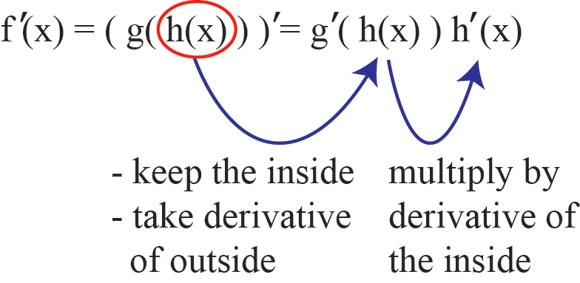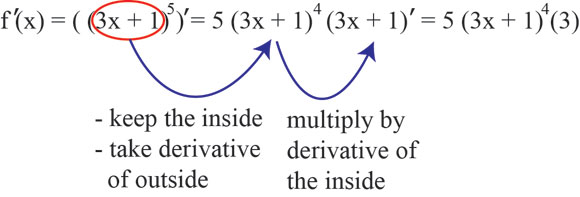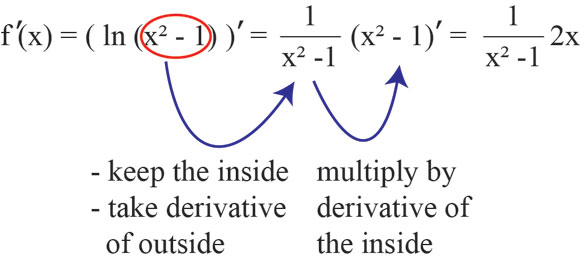﻿ The chain rule - explanation and examples - MathBootCamps

# The chain rule

The chain rule is a method for finding the derivative of composite functions, or functions that are made by combining one or more functions. An example of one of these types of functions is $$f(x) = (1 + x)^2$$ which is formed by taking the function $$1+x$$ and plugging it into the function $$x^2$$. A surprising number of functions can be thought of as composite and the chain rule can be applied to all of them.

## How the formula for the chain rule works

The chain rule says that if $$h$$ and $$g$$ are functions and $$f(x) = g(h(x))$$, thenThis looks complicated, so let’s break it down. The main function $$f(x)$$ is formed by plugging $$h(x)$$ into the function $$g$$. You can think of $$g$$ as the “outside function” and $$h$$ as the “inside function”. Using the chain rule, if you want to find the derivative of the main function $$f(x)$$, you can do this by taking the derivative of the outside function $$g$$ and then multiplying it by the derivative of the inside function $$h$$. In other words, you are finding the derivative of $$f(x)$$ by finding the derivative of its pieces.

## Examples using the chain rule

As we apply the chain rule, we will always focus on figuring out what the “outside” and “inside” functions are first. From there, it is just about going along with the formula.

### Example

Find the derivative of $$f(x) = (3x + 1)^5$$.

### Solution

In this example, there is a function $$3x+1$$ that is being taken to the 5th power. So, there are two pieces: the $$3x + 1$$ (the inside function) and taking that to the 5th power (the outside function). You know by the power rule, that the derivative of $$x^5$$ is $$5x^4$$. So, cover up that $$3x + 1$$, and pretend it is an $$x$$ for a minute. The only deal is, you will have to pay a penalty. Since it was actually not just an $$x$$, you will have to multiply by the derivative of the $$3x+1$$.Simplify to get the final answer:

$$f'(x) = \boxed{5(3x+1)^4(3) = 15(3x+1)^4}$$

### Example

Find the derivative of $$f(x)=\ln(x^2-1)$$.

### Solution

The same idea will work here. Normally, if it was just $$\ln(x)$$, you would say the derivative is $$\dfrac{1}{x}$$. However, there is something there other than $$x$$ (the inside function). So, cover it up and take the derivative anyway. Just don’t forget to multiply by the derivative of the inside function after you are done.Now you can simplify to get the final answer:

$$f'(x)= \boxed{\dfrac{2x}{x^2-1}}$$

If you need to review taking the derivative of ln(x), see this lesson: https://www.mathbootcamps.com/derivative-natural-log-lnx/

## Summary

Composite functions come in all kinds of forms so you must learn to look at functions differently. When trying to decide if the chain rule makes sense for a particular problem, pay attention to functions that have something more complicated than the usual $$x$$. Some examples are $$e^{5x}$$, $$\cos(9x^2)$$, and $$\dfrac{1}{x^2-2x+1}$$. All of these are composite functions and for each of these, the chain rule would be the best approach to finding the derivative.